# 07 - C Loop Control

In this chapter, we will introduce the loop control structure of C language. Looping is a technique through which group of statements are executed repeatedly and it continues execution until that specified condition is reached which decides the termination of loop. This is also called Iterative Mechanism. The specified condition which is responsible for logical test of loop is known as Control statement. When the result for control statement turns out to be false, the loop is terminated. The group of statements is called the body of loop. There are two types of loop control mechanism which are supported in C language and they are as follows:

1.entry-controlled loop

2.exit-controlled loop

Entry controlled loop is the one in which control statement is written before the body of loop. In contrast, exit controlled loop is the one in which control statement is placed after the loop body.

In this chapter, we shall cover the following mechanisms:

• The while statement
• The do-while statement
• the for loop

Let us begin control statements with for loop.

## 7.1The for loop

This is one of thr most popular techniques used by the programmers. To use a for loop we need to specify three things and they are:

• We need to set a loop counter to initial value and this is also known as initialization.
• Control statement is an conditional expression. This performs logical test and the result of this logical test determines the continuation of loop or not.
• Index value which determines increment in loop counter after each repetition. It can be incremented or decremented.

Format of for loop is as follows:Figure-  Format of C for loop

Explanation: Here,

• for is the keyword
• initialization is the initial value to which loop counter is set
• conditional expression is the expression which tests the loop
• increment step value/decrement step value is the value according to which loop counter value is to be incremented/decremented after each repetition.
• statement 1, statement 2, statement 3, etc comprises the body of loop

Example: /* Program to illustrate for loop */

```# include <stdio.h>
# include <conio.h>
# include <math.h>
void main()
{
int number, total:
printf ("This program illustrates the total of 30 numbers\n");
for (number=1; number<=30; number++)
{
total=total+number;
}
printf ("Total=%d\n", total);
getch();
}
```

Snapshots of program is as follows: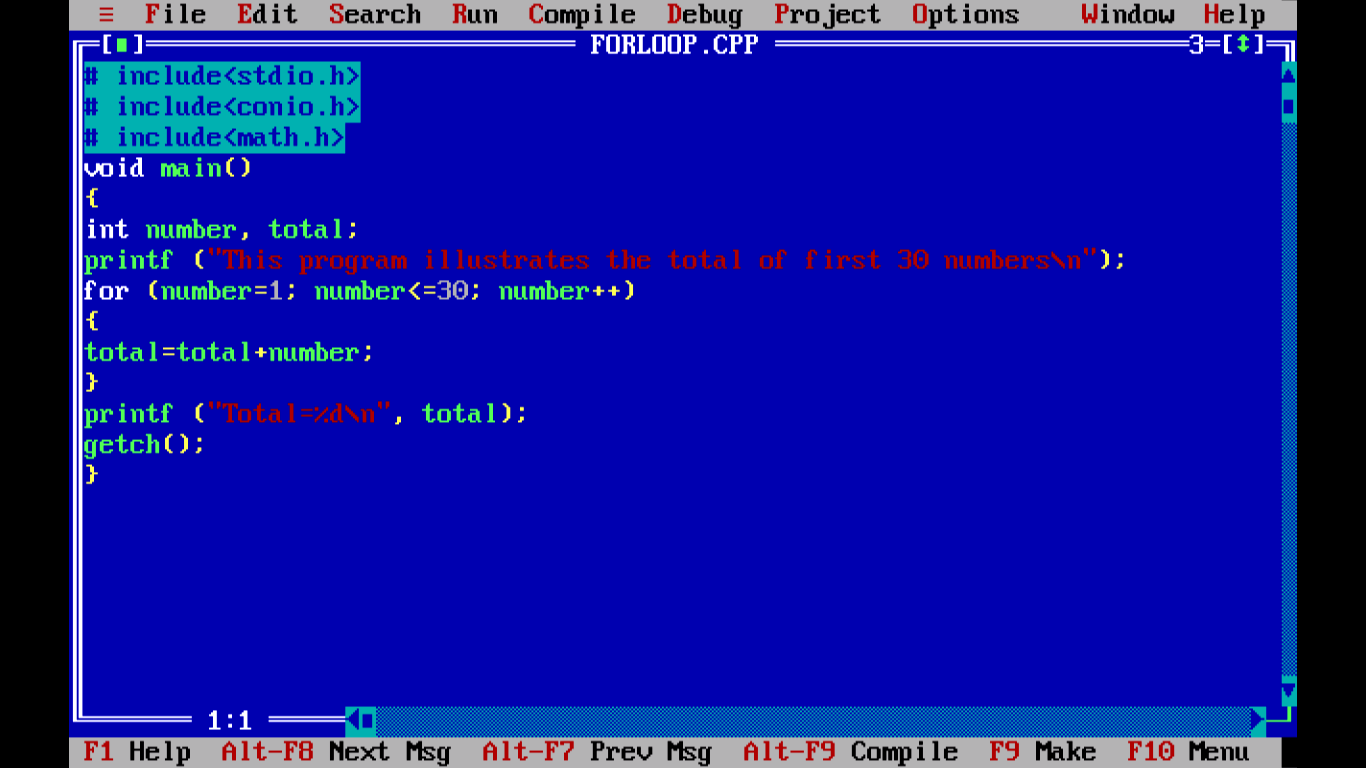Figure - Example of For Loop in C  ProgramFigure - After compiling C program with For Loop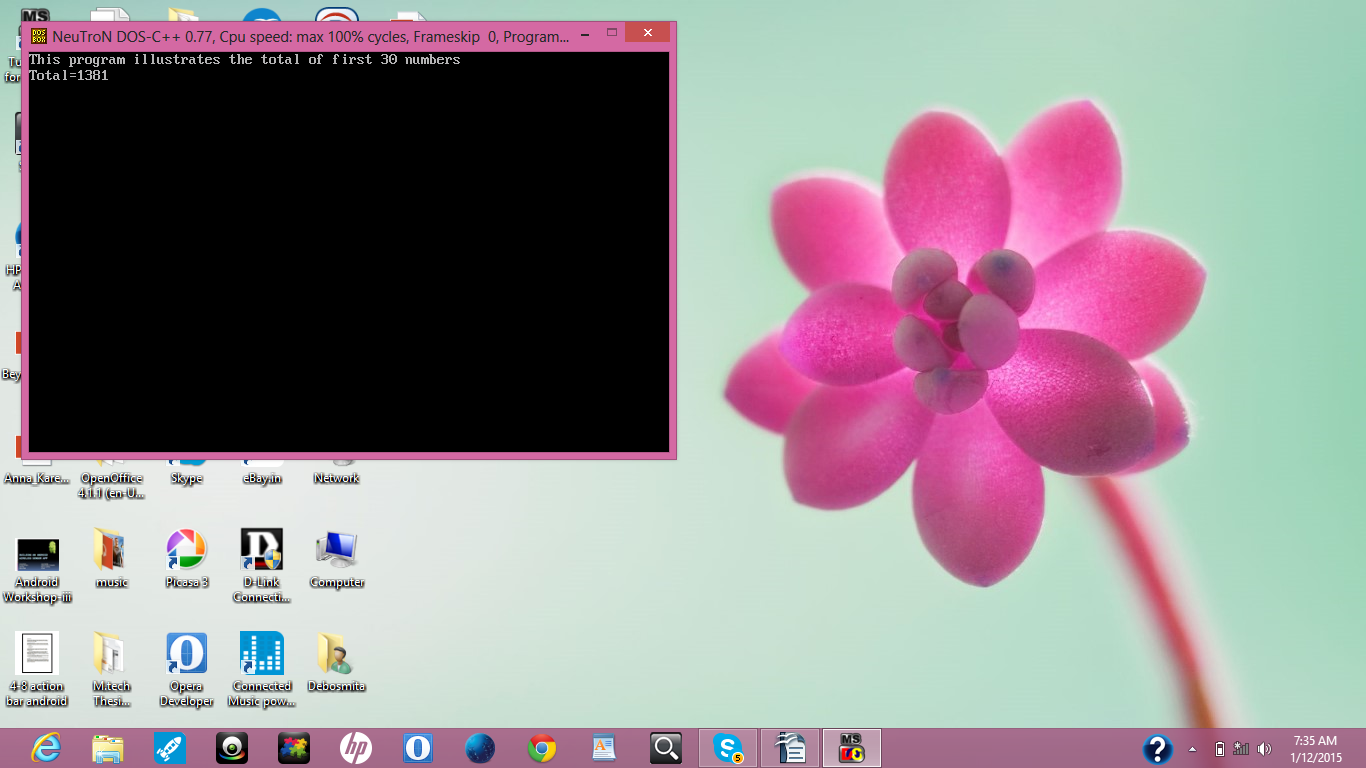Figure - Output of C program with For Loop

The next section describes nested-for loop.

## 7.1.1 Nesting of for loop

There are certain situations where lot of data is to be processed then single for loop is not enough. In this sort of scenarios nesting of for loop is used. Here, one for loop is placed under another for statement. This is known as nested-for loop. Format of nested-for loop is as follows: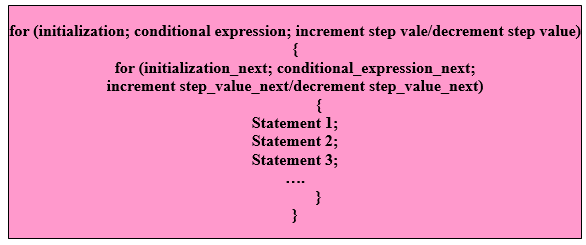Figure - format of nested-for loop

Explanation: Here,

• for is the keyword
• initialization is the initial value to which loop counter is set
• conditional expression is the expression which tests the loop
• step increment/step decrement is the value according to which loop counter value is to be incremented/decremented after each repetition.
• initialization_next is the initial value to which inner for loop counter is set
• conditional_expression_next is the expression which tests the inner for loop
• increment step_value_next/decrement step_value_next is the value according to which loop counter value is to be incremented/decremented after each repetition in inner for loop
• statement 1, statement 2, statement 3, etc comprises the body of loop

Example: /* Program illustrates nested-for loop */

```# include <stdio.h>
# include <conio.h>
# include <math.h>

void main()
{
int number, total:
printf ("This program illustrates the nesting of for loop\n");
for (number=1; number<=5; number++)
{
for (int x=1; x<=4; x++)
{
printf ("Total=%d\n", x);
}
}
getch();
}
```

Snapshots of nested-for loop example program is as follows: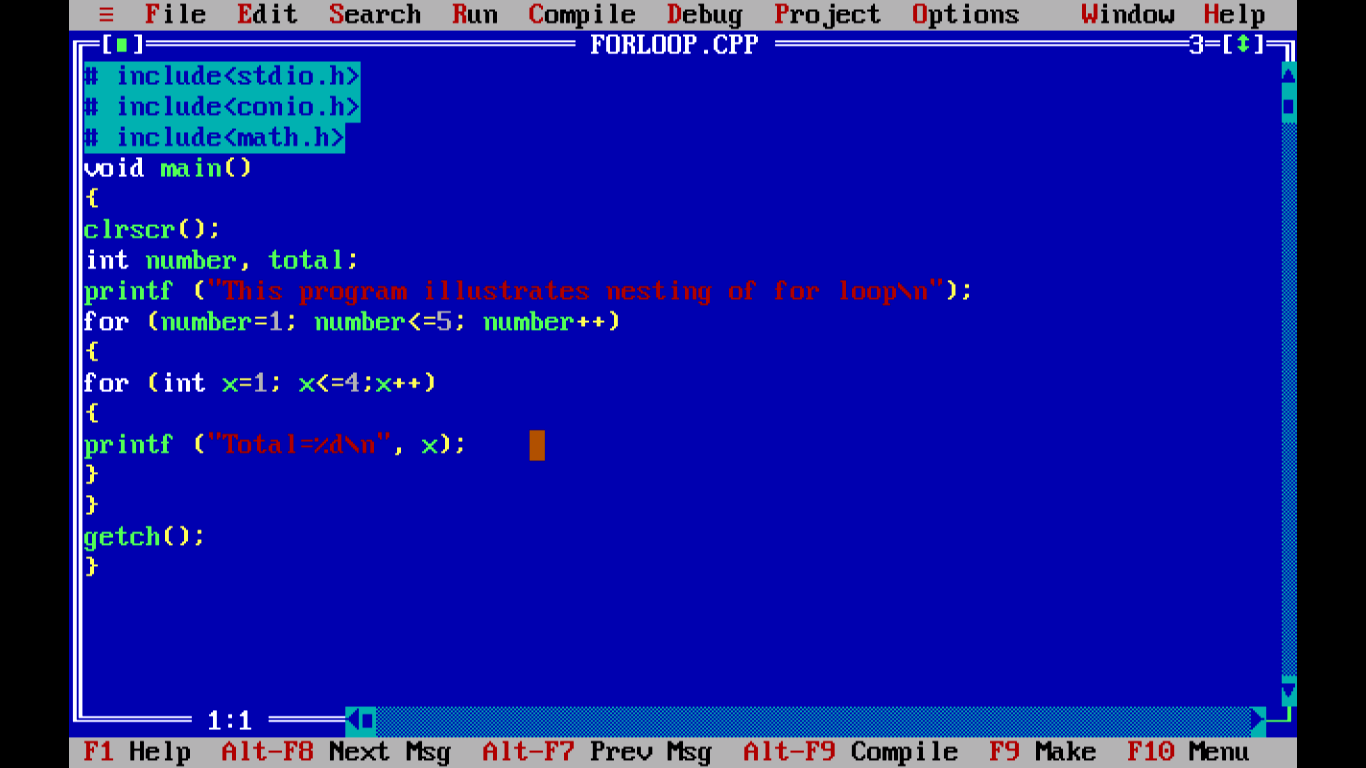Figure - C Program Nested For Loop ExampleFigure - C Program Nested For Loop Example compiled Output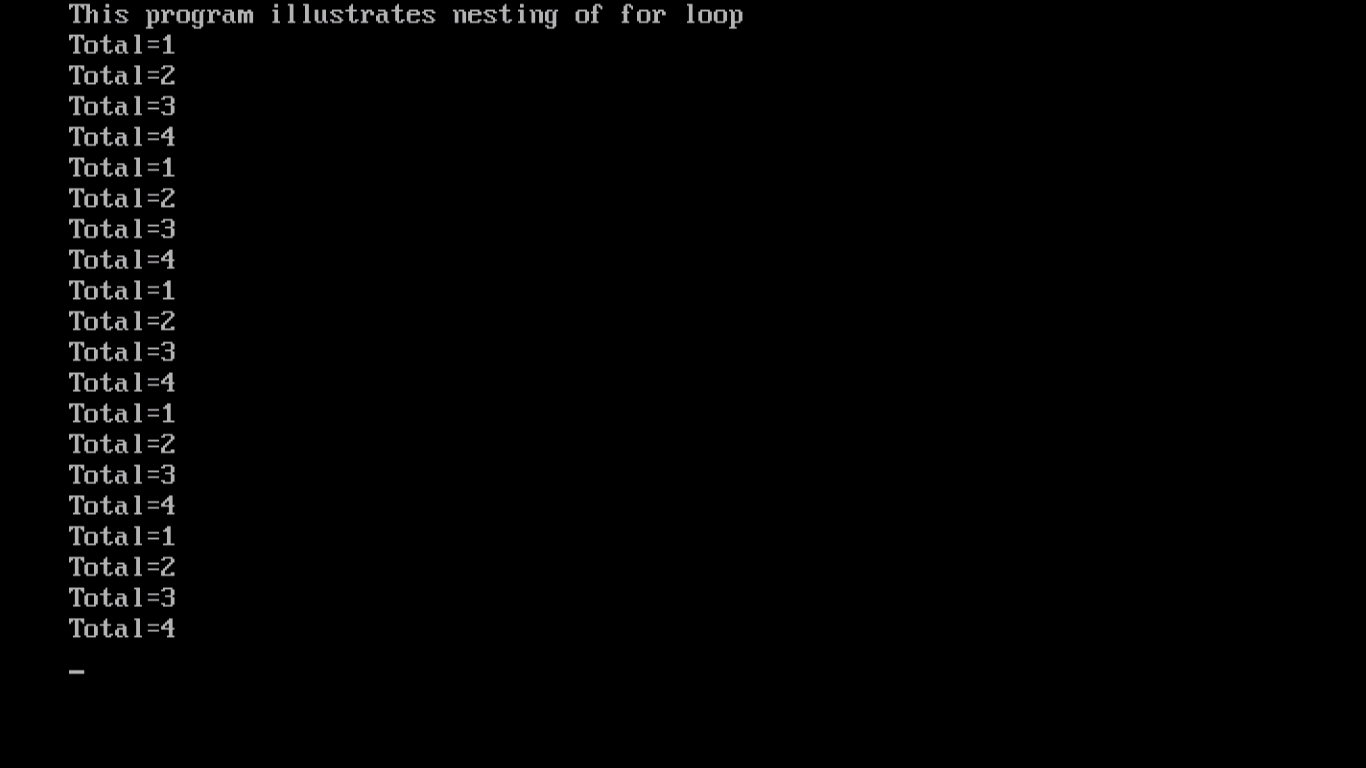Next section will explain while loop.

## 7.2 while loop

While loop executes the encapsulated statements repeatedly until and unless the pretest condition is true. Logical expression is executed first and is test result is true then while is executed. While loop continues to execute till the result is true. As soon as test result turns out to false, control comes out of loop and the statement following while loop is executed. While loop is also known as pretest loop as the result of logical expression always changes at each cycle of execution. As soon as logical expression becomes false control exits the loop. But if the value of logical expression never changes then it enters into an infinite

Format of while loop: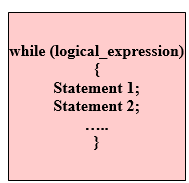Explanation: Here,

• while is the keyword
• logical_expression is a logical expression which is pretested and the result is either a true or false
• statement 1, statement 2, etc is the body of while loop which can contain simple statement or a set of compound statements

Example: /* Program to illustrate while loop */

```# include<stdio.h>
# include <conio.h>
# include <stdlib.h>

void main()
{
clrscr();

char myname;
int times, i=0;

printf("My name is \n");
gets(myname);

printf(" Number of times you want to print your name\n");
scanf("%d", &times);

while(i<times)
{
puts(myname);
i++;
}
getch();
}
```

Snapshots of example program of while loop is as follows: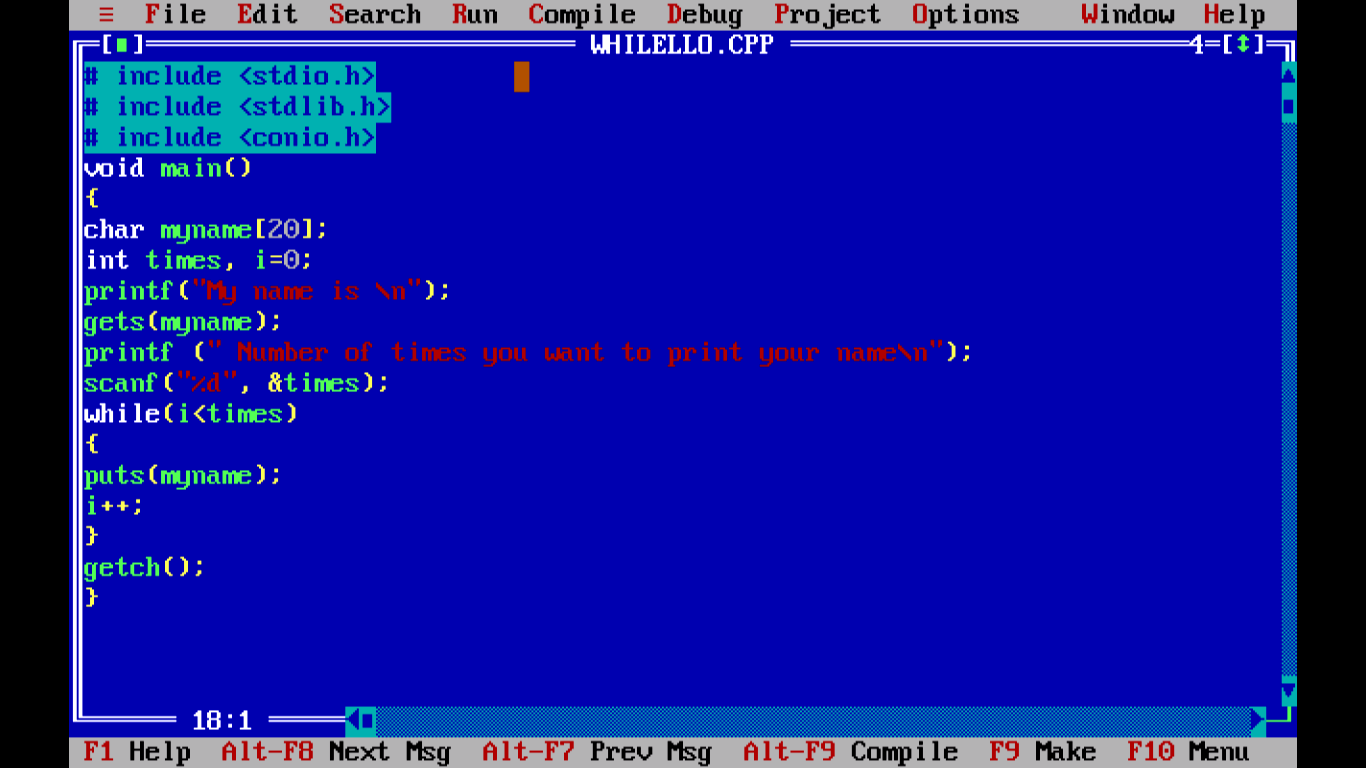Figure - C Program  example with While Loop in editor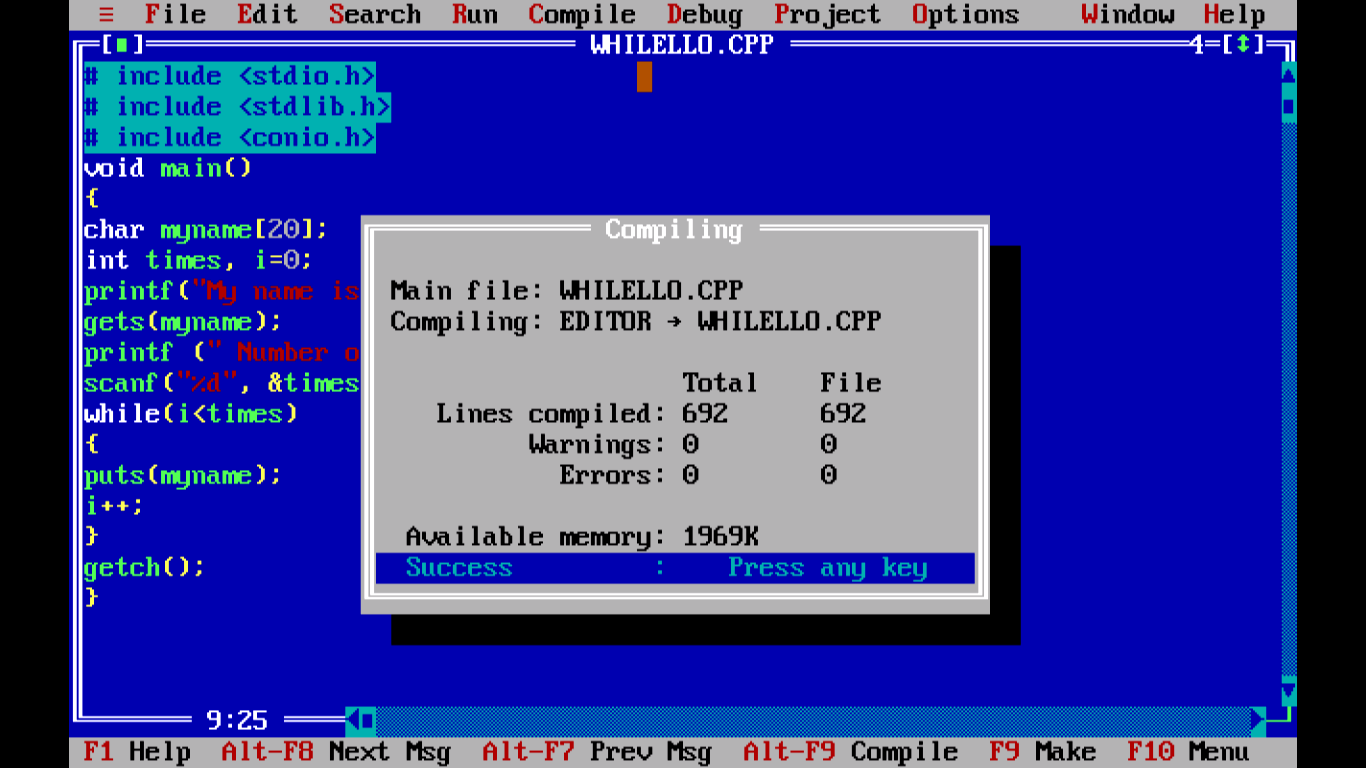Figure - C Program  example with While Loop compiled output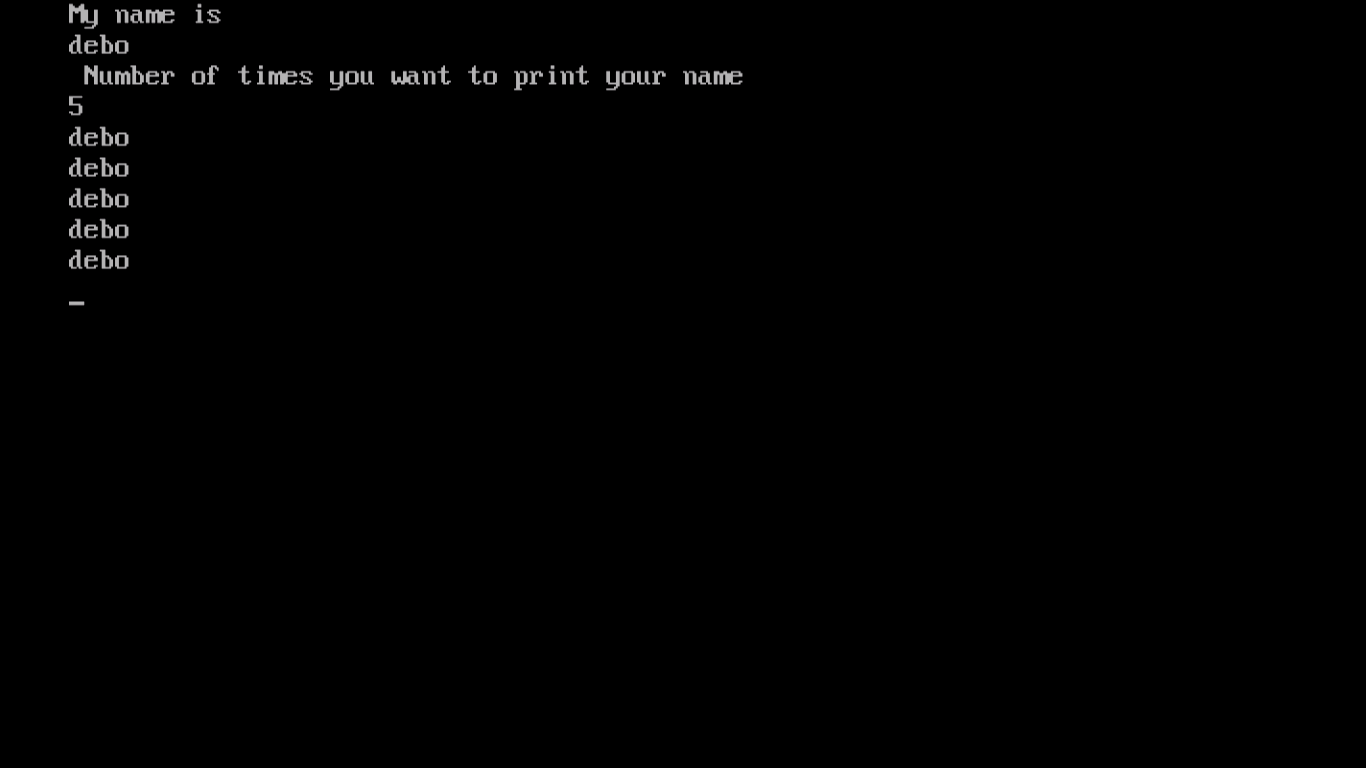Figure -  Output of C Program  example with While Loop

Next section will describe do-while loop.

## 7.3The do-while loop

The do-while loop is similar to while loop. This loop targets at executing set of statements which may be executed repeatedly until a certain condition is reached. There is a minor line of difference between while and do-while loop and that is while loop tests the condition before executing any statements. In contrast, do-while loop tests the condition after executing the statements within while loop. So, at least once the loop is going to be executed. The loop does not execute the statements if test fails. For this particular reason, do-while loop is also known as post-test loop. Format of do-while loop is as follows:

Format of do-while loop:Figure - format of C do-while loop

Explanation: Here,

• do and while are keywords
• logical_expression is the expression which evaluates to either true or false.
• statement 1, statement 2, statement 3, etc comprises the body of while loop.

Example: /* Program to illustrate do-while loop */

```# include <stdio.h>
# include <conio.h>
# include <stdlib.h>

void main()
{
clrscr();
char myname;
int times, i=1;

printf("My name is \n");
gets(myname);

printf(" Number of times you want to print your name\n");
scanf("%d", &times);
puts(myname);
i++;
}while(i<times);

getch();
}
```Figure - C Program with Do-While Loop exampleFigure - C Program compile output with Do-While Loop example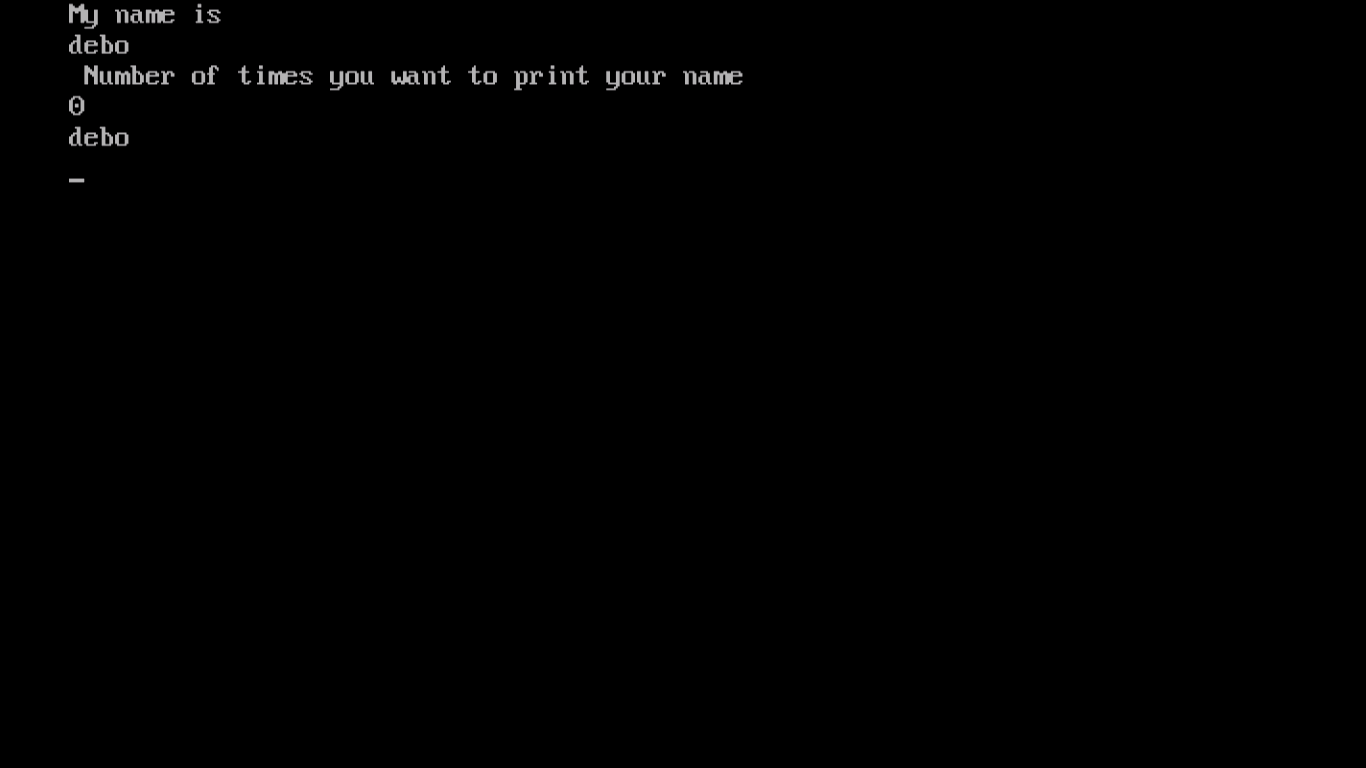Figure - Output of C Program with Do-While Loop example

Next section will describe break statement.

## 7.4 The break statement

The break statement is a weapon in C programming which gives you a way to jump out of loop immediately. Whenever break statement is encountered the control immediately comes out of loop and is transferred to the first statement after the loop. Format of break is as follows:Figure format of C break statement

Explanation: Here,

• break is the keyword

Next section describes continue statement.

## 7.5 The continue statement

The continue statement is like a bypass. Control automatically passes to the beginning of loop and it does not come out of loop like break statement. Format of continue is as follows:Figure - format of C continue statement

Explanation: Here,

• continue is a keyword

With this we conclude this chapter. Next chapter will describe case statement. Thank you.